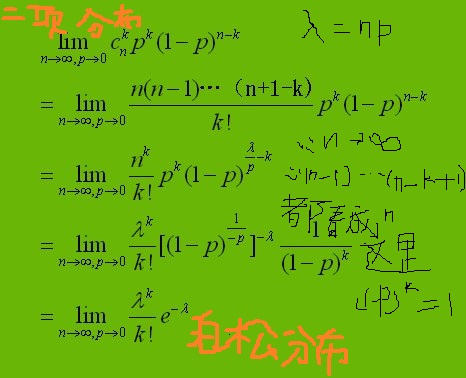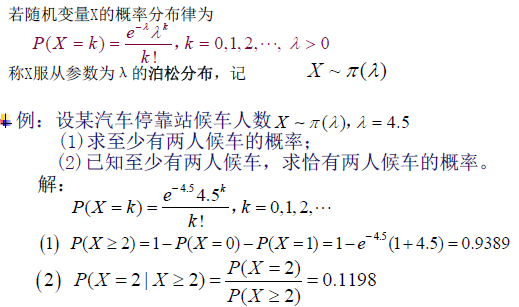( n 中選出 i 項的組合數) * p ^ i * (1-p)^ (n-i)

n * (n-1)... (n-i+1) / i! * p^i * (1-p) ^ (n-i) => np(np-p)...(np-ip+p) / i! * ((1-p) ^ (-1/p))^(-np) / (1-p) ^iLDA的標準過程中單詞出現的次數一般是由泊松分佈來產生的。

﻿﻿
﻿﻿
﻿﻿